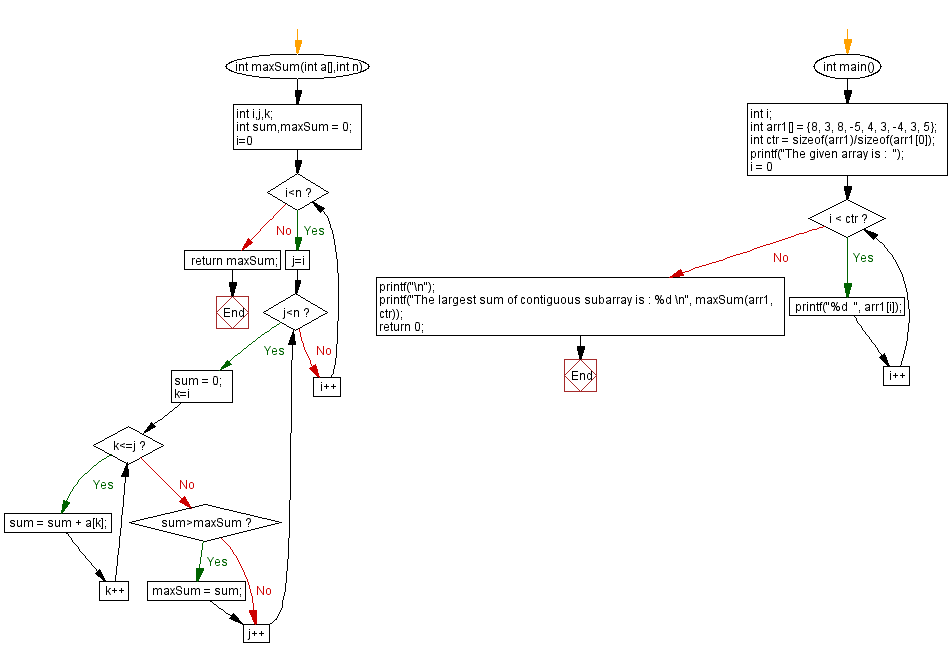﻿ C : Find the largest sum of contiguous subarray of an array

# C Exercises: Find the largest sum of contiguous subarray of an array

## C Array: Exercise-35 with Solution

Write a program in C to find the largest sum of contiguous subarrays in an array.

Sample Solution:

C Code:

``````#include <stdio.h>

int maxSum(int a[],int n)
{
int i,j,k;
int sum,maxSum = 0;
for(i=0; i<n; i++)
{
for(j=i; j<n; j++)
{
sum = 0;
for(k=i ; k<=j; k++)
{
sum = sum + a[k];
}
if(sum>maxSum)
maxSum = sum;
}
}
return maxSum;
}
int main()
{
int i;
int arr1[] = {8, 3, 8, -5, 4, 3, -4, 3, 5};
int ctr = sizeof(arr1)/sizeof(arr1);
printf("The given array is :  ");
for(i = 0; i < ctr; i++)
{
printf("%d  ", arr1[i]);
}
printf("\n");
printf("The largest sum of contiguous subarray is : %d \n", maxSum(arr1, ctr));
return 0;
}
```
```

Sample Output:

```The given array is :  8  3  8  -5  4  3  -4  3  5
The largest sum of contiguous subarray is : 25
```

Flowchart :C Programming Code Editor:

Improve this sample solution and post your code through Disqus.

What is the difficulty level of this exercise?

Test your Programming skills with w3resource's quiz.

﻿

## C Programming: Tips of the Day

What is the static function in C ?

Making a function static hides it from other translation units, which helps provide encapsulation.

helper_file.c

```int f1(int);        /* prototype */
static int f2(int); /* prototype */

int f1(int foo) {
return f2(foo); /* ok, f2 is in the same translation unit */
/* (basically same .c file) as f1         */
}

int f2(int foo) {
return 42 + foo;
}
```

main.c:

```int f1(int); /* prototype */
int f2(int); /* prototype */

int main(void) {
f1(10); /* ok, f1 is visible to the linker */
f2(12); /* nope, f2 is not visible to the linker */
return 0;
}
```

Ref : https://bit.ly/3CI9ebJ

We are closing our Disqus commenting system for some maintenanace issues. You may write to us at reach[at]yahoo[dot]com or visit us at Facebook